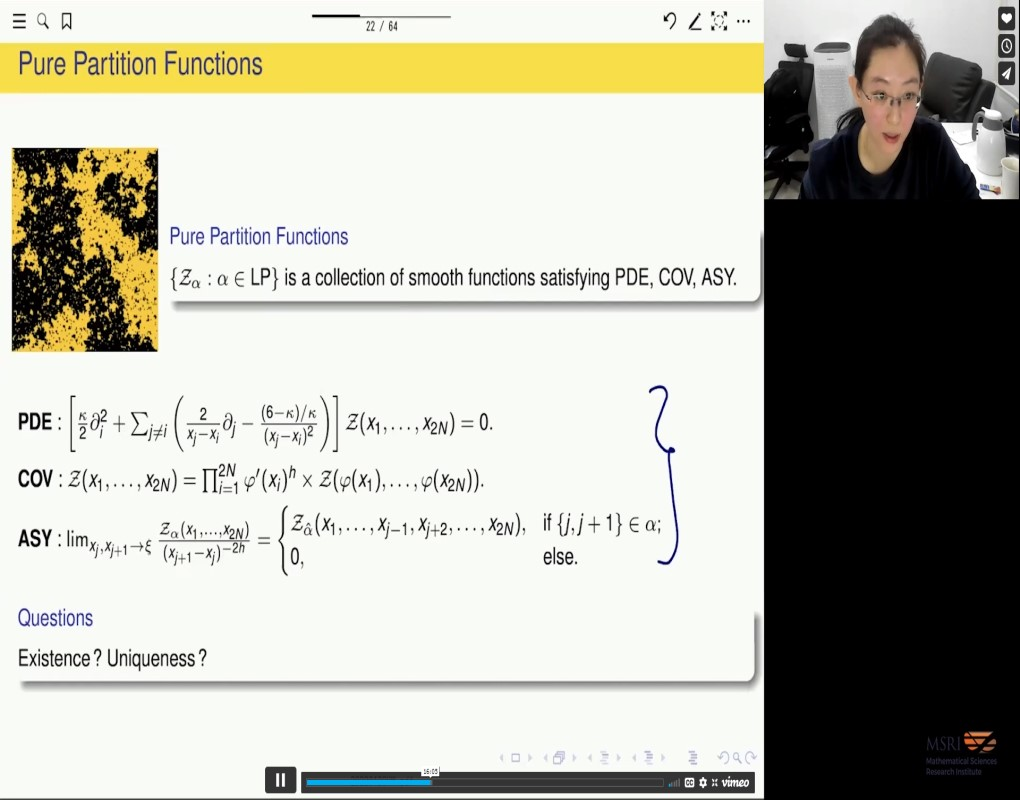### Videos

#### Crossing Probabilities in 2D Critical Lattice Models

##### Presenter
January 19, 2022
Keywords:
• Ising model
• uniform spanning tree
• crossing probabilities
MSC:
• 60J67##### Abstract
Conformal invariance of critical lattice models in two-dimensional has been vigorously studied for decades. The first example where the conformal invariance was rigorously verified was the planar uniform spanning tree (together with loop-erased random walk), proved by Lawler, Schramm and Werner. Later, the conformal invariance was also verified for Bernoulli percolation (Smirnov 2001), level lines of Gaussian free field (Schramm-Sheffield 2009), and Ising model and FK-Ising model (Chelkak-Smirnov et al 2012). In this talk, we focus on crossing probabilities of these critical lattice models in polygons with alternating boundary conditions. The talk has two parts. In the first part, we consider critical Ising model and give crossing probabilities of multiple interfaces in the critical Ising model in polygon with alternating boundary conditions. Similar formulas also hold for other models, for instance level lines of Gaussian free field and Bernoulli percolation. However, the situation is different when one considers uniform spanning tree. In the second part, we discuss uniform spanning tree and explain the corresponding results.